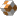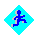# Exploiting Variation for Better Decisions

## Outline.Plan.Concepts.Datasets.Questions.Notes.Summary.

## Plan.

• Provide the flavor and structure of a stat class.
• Introduce ideas and concepts.
• Introduce data.
• Use data to answer interesting questions.
• Summarize.

## Concepts.

• Variability.
• The simple observation that most of the data we record is variable -- not constant.
• We need to measure/quantify the variabilty in order to exploit it.
• Understanding variability/precision is what differentiates statistical from other quantitative methodologies.
• Graphical approach to analyzing data.
• Dynamic graphics.
• Emphasize interpretation, not math.

## Data sets.gm92.jmp Daily returns on General Motors, 1992-93.gmat.jmp Gmat scores of the class of 94.

## Questions.

• How can we use the statistical summary of the data and a probability model to measure risk?
• Were all MBA students subject to the same admission criteria?

## Notes.

• Smoothing.
• Looking for structure.
• Normal model.
• Empirical rule. 95% of data lies within +/- 2 s.d. of the mean.
• 95% of the data lies to the right of 1.645 s.d. below the mean.
• Crude VaR calculation. If mean about 0, just do 1.645 s.d. below the mean.
• For GM get VaR on \$100.00 = \$100 * 1.645 * 0.02 = \$3.13.

## Summary.

• Condensed stat class.
• Interpretative, conceptual and graphical emphasis.
• Application of statistical ideas in a business context.